Publication date: 11/10/2021

## Estimation Efficiency

This report gives the Fractional Increase in CI (Confidence Interval) Length and Relative Std (Standard) Error of Estimate for each parameter estimate in the model. Smaller is better for both of these values. Figure 15.22 shows the Estimation Efficiency outline for the Bounce Data.jmp sample data table, located in the Design Experiment folder.

Figure 15.22 Estimation Efficiency Outline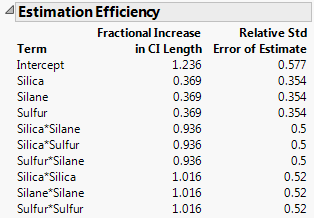### Fractional Increase in CI Length

The Fractional Increase in CI Length compares the length of a parameter’s confidence interval as given by the current design to the length of such an interval given an ideal design:

The length of the ideal confidence interval for the parameter is subtracted from the length of its actual confidence interval.

This difference is then divided by the length of the ideal confidence interval.

For an orthogonal D-optimal design, the fractional increase is zero. In selecting a design, you would like the fractional increase in confidence interval length to be as small as possible.

#### The Ideal Design

The covariance matrix for the ordinary least squares estimator is σ2(XX)-1. The diagonal elements of (XX)-1 are the relative variances (the variances divided by σ2) of the parameter estimates. For two-level designs and using the effects coding convention (see Coding), the minimum value of the relative variance for any parameter estimate is 1/n, where n is the number of runs. This occurs when all the effects for the design are orthogonal and the design is D-optimal.

Let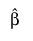denote the vector of parameter estimates. The ideal design, which may not exist, is a design whose covariance matrix is given as follows: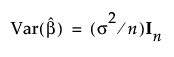where In is the n by n identity matrix and σ is the standard deviation of the response.

If an orthogonal D-optimal design exists, it is the ideal design. However, the definition above extends the idea of an ideal design to situations where a design that is both orthogonal and D-optimal does not exist.

The definition is also appropriate for designs with multi-level categorical factors. The orthogonal coding used for categorical factors allows such designs to have the ideal covariance matrix. For a Custom Design, you can view the coding matrix by selecting Save X Matrix from the options in the Custom Design window, making the design table, and looking at the script Model Matrix that is saved to the design table.

#### Fractional Increase in Length of Confidence Interval

Note that, in the ideal design, the standard error for the parameter estimates would be given as follows: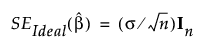The length of a confidence interval is determined by the standard error. The Fractional Increase in Confidence Interval Length is the difference between the standard error of the given design and the standard error of the ideal design, divided by the standard error of the ideal design.

Specifically, for the ith parameter estimate, the Fractional Increase in Confidence Interval Length is defined as follows: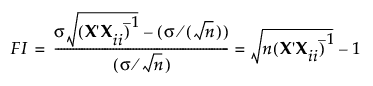where

σ2 is the unknown response variance,

X is the model matrix for the given design, defined in The Alias Matrix,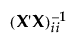is the ith diagonal entry of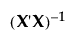, and

n is the number of runs.

### Relative Std Error of Estimate

The Relative Std Error of Estimate gives the ratio of the standard deviation of a parameter’s estimate to the error standard deviation. These values indicate how large the standard errors of the model’s parameter estimates are, relative to the error standard deviation. For the ith parameter estimate, the Relative Std Error of Estimate is defined as follows: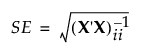where

X is the model matrix defined in The Alias Matrix, andis the ith diagonal entry of.